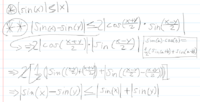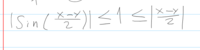# Absolute value of sin(x)

#### Wernilou

##### New member
Hey, first post here, with all the world crisis rn, we didn't have a final test but a final project, a 3 page project, and i'm horrible at this. I can't even solve the first one, which is this, please i need some help.

Use the that |sin(x)|≤|x| and the formula that makes sin(x)-sin(y) in a product to demonstrate that |sin(x)-sin(y)|≤|x-y|

This is what i have, but i can't go further, I'm desperate, i will be even more desperate because the work has 11 problems that subdivide, and I am a total useless, so please if someone could help me only with this one#### Dr.Peterson

##### Elite Member
I think you're making it much more complicated than it is. You haven't used the given fact (*)!

I probably shouldn't be helping much at all for a graded project, but I'll just suggest that you scratch the last three lines and think.

(Also, be more careful about your inequalities and implications; the line (**) could be an equality!)

•Wernilou

#### Jomo

##### Elite Member
$$\displaystyle \dfrac{sin(x) - sin(y)}{x-y}$$ looks so much like a derivative to me. So maybe you could use that to make some progress.

•Wernilou

#### Wernilou

##### New member
I think you're making it much more complicated than it is. You haven't used the given fact (*)!

I probably shouldn't be helping much at all for a graded project, but I'll just suggest that you scratch the last three lines and think.

(Also, be more careful about your inequalities and implications; the line (**) could be an equality!)
I really don't know how to continue with that, it breaks my head, i can't just add sin(y)< y to it, i dont know what to do after that

#### Dr.Peterson

##### Elite Member
It's telling you that |sin(x)|≤|x| for all x. So you can replace x in that statement with whatever you feel like.

#### Dr.Peterson

##### Elite Member
Look at your line marked (**). I said that entire line works in the right direction. Try a different substitution, not x-y.

A general principle in proofs is to try anything, and see what happens. With practice, you can learn to recognize unfruitful paths a little more quickly; but you may still need to try things that don't immediately look helpful. So, what else do you see as the argument of a sine? Try it!

#### Wernilou

##### New member
Look at your line marked (**). I said that entire line works in the right direction. Try a different substitution, not x-y.

A general principle in proofs is to try anything, and see what happens. With practice, you can learn to recognize unfruitful paths a little more quickly; but you may still need to try things that don't immediately look helpful. So, what else do you see as the argument of a sine? Try it!this?, i don't know how to clean that?

#### pka

##### Elite Member
Use the that |sin(x)|≤|x| and the formula that makes sin(x)-sin(y) in a product to demonstrate that |sin(x)-sin(y)|≤|x-y|
I have no idea what your author is aiming at with the direction "Use the that |sin(x)|≤|x|".
However this is a well-known exercise that applies the mean value theorem.
Consider the function $$f(x)=\sin(x)$$ on the interval $$[x,y];~x<y$$.
Then $$\exists c\in(x,y)$$ so that $$\cos(c)=\dfrac{f(y)-f(x)}{y-x}$$
Can you see how to finish?

#### Dr.Peterson

##### Elite Member
View attachment 17438this?, i don't know how to clean that?
Put this together with the rest of what you've done.

But as Jomo and pka pointed out, this can be approached in many ways, and we are at a disadvantage in not knowing what you have learned. The approach I am trying to follow, based on what was said in the problem, is not very closely related to calculus.

#### firemath

##### Full Member
I also was a bit confused at the wording of the problem. Could we have the exact problem?

#### Jomo

##### Elite Member
View attachment 17438this?, i don't know how to clean that?
So matter what x and y I pick (x-y)/2 is always at least 1? You know that is not true. Suppose x=15 and y =14, then (x-y)/2 = 1/2 which is less than 1.

Last edited:

#### Jomo

##### Elite Member
Lets us use what you were given and want to show.

You want to show that |sin(x) - sin(y)| < |x-y|. It was suggested that you should use the fact that sin(x) - sin(y) = 2cos[(x+y)/2]sin[(x-y)/2]

The hint is telling you that instead of showing that sin(x)-sin(y)| < |x-y| you should instead show that |2cos[(x+y)/2]sin[(x-y)/2]| < |x-y|

Do you agree that showing that |sin[(x+y)/2]cos[(x-y)/2]| < |x-y|/2 is the same as sin(x)-sin(y)| < |x-y| ??

Can the following help? I would definitely try this but in the end it might not help. It is a classic substitution in proving other trig identities. Let a = (x+y)/2 and b = (x-y)/2. Adding yields that a+b = x and a-b =y

#### Wernilou

##### New member
Lets us use what you were given and want to show.

You want to show that |sin(x) - sin(y)| < |x-y|. It was suggested that you should use the fact that sin(x) - sin(y) = 2cos[(x+y)/2]sin[(x-y)/2]

The hint is telling you that instead of showing that sin(x)-sin(y)| < |x-y| you should instead show that |2cos[(x+y)/2]sin[(x-y)/2]| < |x-y|

Do you agree that showing that |sin[(x+y)/2]cos[(x-y)/2]| < |x-y|/2 is the same as sin(x)-sin(y)| < |x-y| ??

Can the following help? I would definitely try this but in the end it might not help. It is a classic substitution in proving other trig identities. Let a = (x+y)/2 and b = (x-y)/2. Adding yields that a+b = x and a-b =y
I don't understand how that inequality would be proven...

#### Dr.Peterson

##### Elite Member
The hint is telling you that instead of showing that sin(x)-sin(y)| < |x-y| you should instead show that |2cos[(x+y)/2]sin[(x-y)/2]| < |x-y|

Do you agree that showing that |sin[(x+y)/2]cos[(x-y)/2]| < |x-y|/2 is the same as sin(x)-sin(y)| < |x-y| ??

Can the following help? I would definitely try this but in the end it might not help. It is a classic substitution in proving other trig identities. Let a = (x+y)/2 and b = (x-y)/2. Adding yields that a+b = x and a-b =y
I don't understand how that inequality would be proven...
First, I notice that Jomo got some signs wrong. Check that.

What do you know to be true of |sin[(x-y)/2]|, based on the stated fact that |sin(a)|≤|a| for all a?

What do you know to be true of |cos[(x+y)/2]| ? Keep it simple.

What does that tell you about |2cos[(x+y)/2]sin[(x-y)/2]| ?

What does that tell you about |sin(x)-sin(y)| ?

#### Wernilou

##### New member
First, I notice that Jomo got some signs wrong. Check that.

What do you know to be true of |sin[(x-y)/2]|, based on the stated fact that |sin(a)|≤|a| for all a?

What do you know to be true of |cos[(x+y)/2]| ? Keep it simple.

What does that tell you about |2cos[(x+y)/2]sin[(x-y)/2]| ?

What does that tell you about |sin(x)-sin(y)| ?
that sin(x-y/2) ≤ x-y/2
that |cos[(x+y)/2]| ≤ 1
i dont know....

#### Dr.Peterson

##### Elite Member
that sin(x-y/2) ≤ x-y/2
that |cos[(x+y)/2]| ≤ 1
i dont know....
Don't forget the parentheses, without which that doesn't mean what you intend!

Then ... this is your final project, so I expect you to complete it, so that you can say that you succeeded. Just think.

You're very close, so just open your eyes and relate what you just said to what you already said, and to what the goal is.

#### Wernilou

##### New member
Can't really finish it, so rip

#### Dr.Peterson

##### Elite Member
You can lead a student to knowledge, but you can't make them think ...

I'll give it one more try.

You know that $$\displaystyle |\sin(x) - \sin(y)| = 2\left|\cos\left(\frac{x+y}{2}\right)\sin\left(\frac{x-y}{2}\right)\right|$$.

You know that $$\displaystyle \left|\cos\left(\frac{x+y}{2}\right)\right| \le 1$$.

You know that $$\displaystyle \left|\sin\left(\frac{x-y}{2}\right)\right|\le \left|\frac{x-y}{2}\right|$$.

Put those three things, all of which you yourself have said, together!